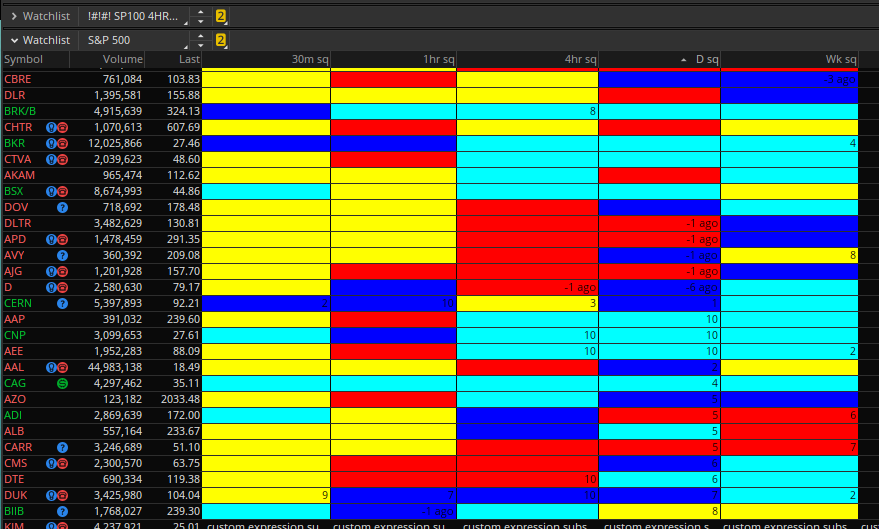# Squeeze Watchlist Column - Histrogram Colors, For ThinkOrSwim

#### dunpeal

##### New member
Has histo colors, squeeze count, and how long ago a squeeze fired. I like ordering the column numerically as you can see in the Daily. Now I can watch whatever time frame and see what has fired. You can look left on the small time frames and get an idea of which direction it fired. Exampled is DLTR, fired on the daily and went down on the 30m,1hr,4hr.

DUK will be an interesting one coming up with earnings.Code:
``````input price = CLOSE;
input length = 20;
input nK = 1.5;
input nBB = 2.0;
def keltnerChannelATRs = 1.5 ;
def bollingerBandStdDev = 2 ;

# Average True Range
def ATR = Average(TrueRange(high,  close,  low),  20);

# Standard Deviation
def SDev = StDev(close, 20);

# -- Calculate Bollinger Band Squeeze Indicator --
def Denom = (keltnerChannelATRs * ATR);
def BBS_Ind = If (Denom <> 0, ((bollingerBandStdDev * SDev) / Denom), 0);#A squeeze is on if BBS_Ind < 1.0

def squeeze = if TTM_Squeeze().SqueezeAlert == 0 then 1 else 0;
def sumSqueeze = Sum(squeeze, 10);
def squeezeFired = if TTM_Squeeze().SqueezeAlert == 0 and TTM_Squeeze(). SqueezeAlert == 1 then 1 else 0;
AddLabel (!squeezeFired and !squeeze, " ", color.black);
def squeezeHistogram = TTM_Squeeze(price, length, nK, nBB, alertLine).Histogram;
plot hist = if squeezeHistogram>= 0 then
if squeezeHistogram> squeezeHistogram then 2 else 1
else if squeezeHistogram < squeezeHistogram then -2 else -1; assignBackgroundColor(if squeezeHistogram >= 0 then
if squeezeHistogram > squeezeHistogram then color.CYAN else color.BLUE

else if squeezeHistogram < squeezeHistogram then color.RED
else color.YELLOW); hist.assignvaluecolor(if squeezeHistogram >= 0 then
if squeezeHistogram > squeezeHistogram then color.CYAN else color.BLUE

else if squeezeHistogram < squeezeHistogram then color.RED
else color.YELLOW);

#### How long ago the squeeze fired
def Post_Count = if BBS_Ind < 1.0  && BBS_Ind > 1.0 then 1
else if BBS_Ind < 1.0  && BBS_Ind > 1.0 then 2
else if BBS_Ind < 1.0  && BBS_Ind > 1.0 then 3
else if BBS_Ind < 1.0  && BBS_Ind > 1.0 then 4
else if BBS_Ind < 1.0 && BBS_Ind > 1.0  then 5
else if BBS_Ind < 1.0 && BBS_Ind > 1.0 then 6
else Double.NaN;

AddLabel (Post_Count,Concat("-", Concat(Post_Count, " ago")), color.BLACK);
AddLabel (!squeezeFired and !squeeze, " ", color.black);``````
https://tos.mx/UA6QBwB

WOW thatk you for sharring

@dunpeal thanks for the watchlist !! i found it very helpful. I have question. Is it possible to add the squeese and presqueeze count with this ttm pro watchlist found on this forum. Thank you!

input price = close;
input length = 20;
input Num_Dev_Dn = -2.0;
input Num_Dev_up = 2.0;
input averageType = AverageType.SIMPLE;
input displace = 0;
def sDev = StDev(data = price[-displace], length = length);
def MidLineBB = MovingAverage(averageType, data = price[-displace], length = length);
def LowerBandBB = MidLineBB + Num_Dev_Dn * sDev;
def UpperBandBB = MidLineBB + Num_Dev_up * sDev;
input factorhigh = 1.0;
input factormid = 1.5;
input factorlow = 2.0;
input trueRangeAverageType = AverageType.SIMPLE;
def shifthigh = factorhigh * MovingAverage(trueRangeAverageType, TrueRange(high, close, low), length);
def shiftMid = factormid * MovingAverage(trueRangeAverageType, TrueRange(high, close, low), length);
def shiftlow = factorlow * MovingAverage(trueRangeAverageType, TrueRange(high, close, low), length);
def average = MovingAverage(averageType, price, length);

def Avg = average[-displace];

def UpperBandKCLow = average[-displace] + shiftlow[-displace];
def LowerBandKCLow = average[-displace] - shiftlow[-displace];

def UpperBandKCMid = average[-displace] + shiftMid[-displace];
def LowerBandKCMid = average[-displace] - shiftMid[-displace];

def UpperBandKCHigh = average[-displace] + shifthigh[-displace];
def LowerBandKCHigh = average[-displace] - shifthigh[-displace];

def K = (Highest(high, length) + Lowest(low, length)) /
2 + ExpAverage(close, length);
def momo = Inertia(price - K / 2, length);

def pos = momo>= 0;
def neg = momo< 0;
def up = momo >= momo;
def dn = momo < momo;

def presqueeze = LowerBandBB > LowerBandKCLow and UpperBandBB < UpperBandKCLow;
def originalSqueeze = LowerBandBB > LowerBandKCMid and UpperBandBB < UpperBandKCMid;
def ExtrSqueeze = LowerBandBB > LowerBandKCHigh and UpperBandBB < UpperBandKCHigh;

addlabel(yes, if extrSqueeze then "extraSqueeze" else if originalSqueeze then "squeeze" else if presqueeze then "preSqueeze" else "noSqueeze");
assignBackgroundColor(if ExtrSqueeze then Color.red else if originalSqueeze then Color.blue else if presqueeze then color.white else Color.gray);

•Topcat357

### Not the exact question you're looking for?

87k+ Posts
169 Online## The Market Trading Game Changer

Join 2,500+ subscribers inside the useThinkScript VIP Membership Club
• Exclusive indicators
• Proven strategies & setups
• Private Discord community
• Exclusive members-only content
• 1 full year of unlimited support

What is useThinkScript?

useThinkScript is the #1 community of stock market investors using indicators and other tools to power their trading strategies. Traders of all skill levels use our forums to learn about scripting and indicators, help each other, and discover new ways to gain an edge in the markets.

How do I get started?

We get it. Our forum can be intimidating, if not overwhelming. With thousands of topics, tens of thousands of posts, our community has created an incredibly deep knowledge base for stock traders. No one can ever exhaust every resource provided on our site.

If you are new, or just looking for guidance, here are some helpful links to get you started.

What are the benefits of VIP Membership?